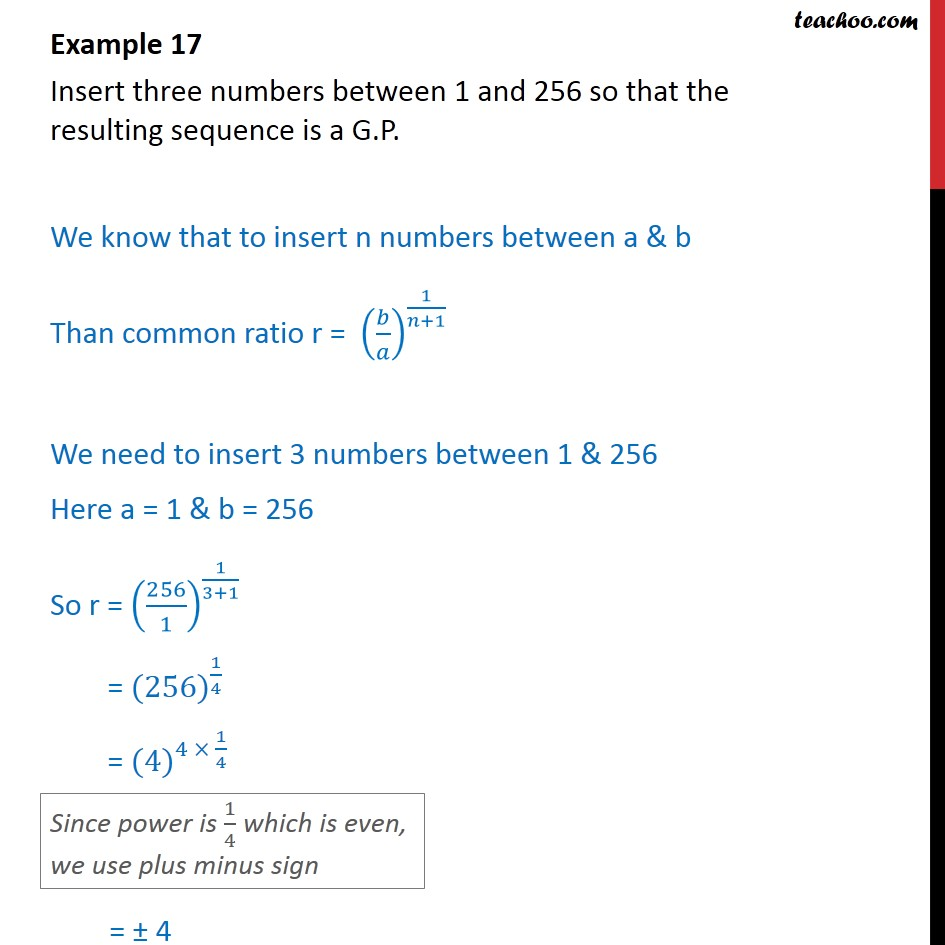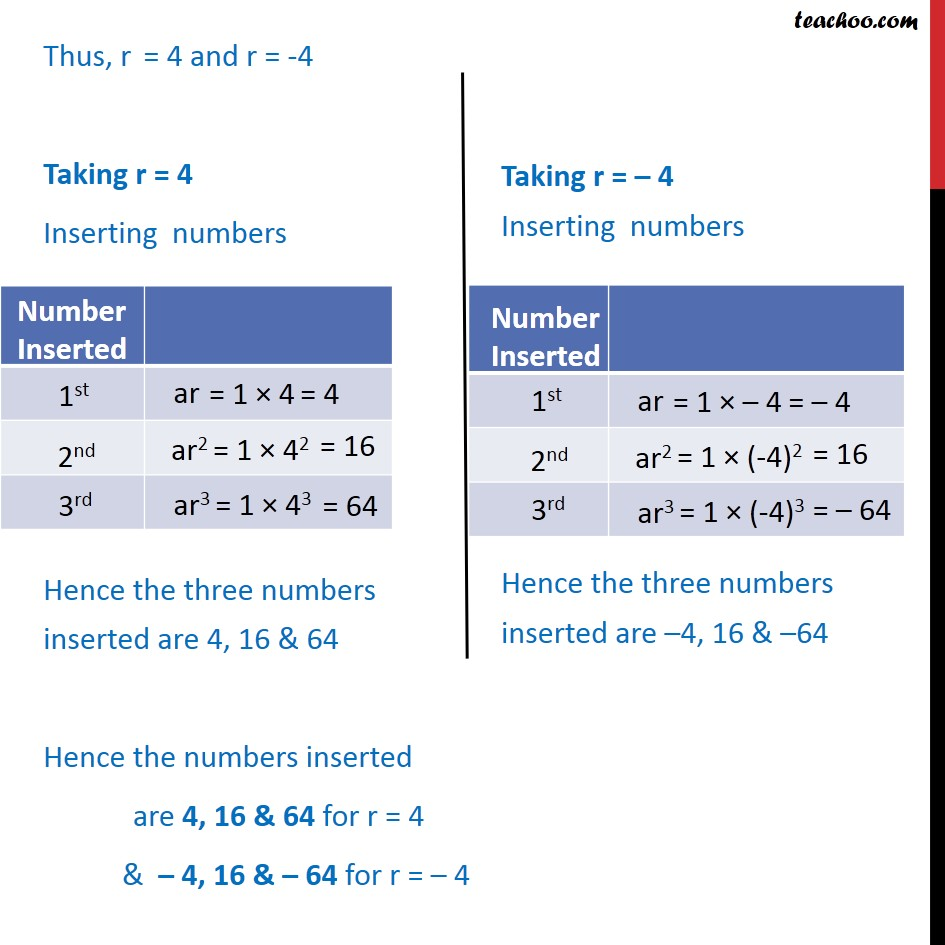Examples

Chapter 8 Class 11 Sequences and Series
Serial order wiseLearn in your speed, with individual attention - Teachoo Maths 1-on-1 Class

### Transcript

Example 12 Insert three numbers between 1 and 256 so that the resulting sequence is a G.P. We know that to insert n numbers between a & b Than common ratio r = 1 +1 We need to insert 3 numbers between 1 & 256 Here a = 1 & b = 256 So r = 256 1 1 3+1 = (256) 1 4 = (4) 4 1 4 = 4 Thus, r = 4 and r = -4 Taking r = 4 Inserting numbers Hence the three numbers inserted are 4, 16 & 64 Hence the numbers inserted are 4, 16 & 64 for r = 4 & 4, 16 & 64 for r = 4